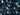# Many qubits and entangled statesIn quantum computing, the idea of many qubits and entangled states describes the interaction and information sharing between more than two quantum bits (qubits). A quantum bit, or qubit, is a unit of quantum information that may simultaneously take on the values 0 and 1, among others, in the classical world. Entanglement between two qubits means that their states are mutually reliant and cannot be described separately. As a quantum phenomenon, entanglement allows quantum computing to accomplish goals that would be impossible or inefficient for classical computing.

Here are a few functions that can be performed on multiple qubits:

Mathematically, two qubits may be combined into a four-dimensional vector that describes their combined state through the tensor product. Logic gates that operate on one or more qubits are called quantum gates, and examples include the CNOT gate, which flips the state of one qubit based on the state of another. We can execute computations on numerous qubits using quantum circuits, which are sequences of quantum gates. By use of a coupler device, such as a superconducting flux qutrit, the quantum state of one kind of qubit may be transferred to another type of qubit, such as from a single-photon state qubit to a coherent-state qubit. Due of their ability to facilitate parallel processing, interference, and error correction, quantum computers cannot function without several qubits and entangled states. They also pave the way for useful technologies like quantum cryptography, teleportation, and measurement equipment.

XPERIMEN.COM